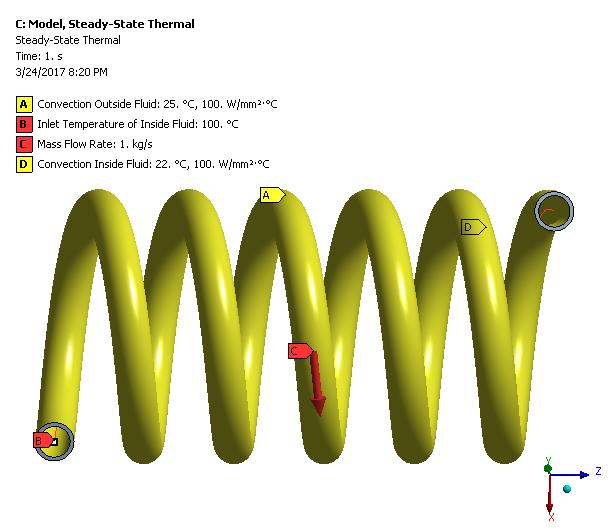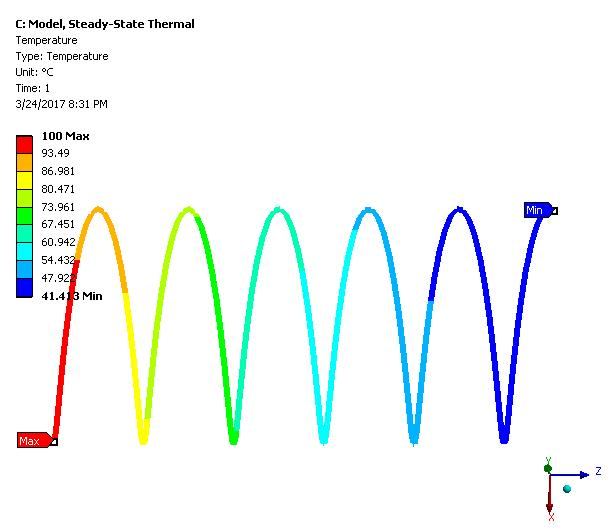## Fluids

Topics relate to Fluent, CFX, Turbogrid and more

•peteroznewman
Subscriber

I was interested in a model to evaluate the temperature change of water flowing through a tube immersed in a tank of water.  I modeled 5 turns of a helix with a radius of 30 mm, pitch of 23 mm, aluminum tube OD of 10 mm with a 1 mm wall thickness. I used Excel to create a file of points on the helix and imported that file into DesignModeler as a 3D curve.  A line body represents the water flowing in the tube. The flow rate is 1 kg/s and the input temperature is 100 C. A solid body represents the tube. A convection BC represents the outside of the tube losing heat to the outside water at a temperature of 25 C. A second convection BC represents the inside of the tube taking heat from the fluid inside the tube.After I solved this system, I plotted the temperature of the water in the tube.I was interested to learn that I could obtain results from the Steady State Thermal system block that I initially thought I would have to build a much more complicated CFD model to get similar results.

•Raef.Kobeissi
Subscriber
A great example to share!
•peteroznewman
Subscriber

Thanks Raef.

The above model was in response to a student who asked how to create a mesh for a helical coil to do convective heat transfer. Upon further correspondence, I learned his project was to build skill in CFD by reproducing a model described in a research paper

After I read that paper, I understood that rather than being given the convective heat transfer coefficient, which I needed in the above model as an input, the purpose was to build a CFD model to calculate the convective heat transfer coefficient as a result from the simulation. This was something that interested me also.

Cheers,
Peter